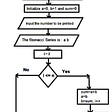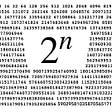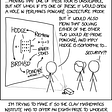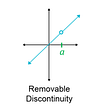# Part 5: Revisiting Systems of Linear Equations

## Delving deeper into row operations

In part 1, I explored how matrices can be used to solve systems of linear equations. I talked about row operations, matrices, REF and RREF form, and other key concepts that can help us solve systems of equations using linear algebra. However, I didn’t actually hit on the mechanics of row operations, and so after reading and learning about how they work, I am proud to say that I can now explain row operations in this article right here. I’ll go over what row operations entail, the methods for reducing a matrix into its reduced row echelon form (RREF), and the different ties back to the systems of linear equations that we want to solve in the first place.

## What are Row Operations?

In my own words, row operations are certain “altercations” that we can make to matrices which can drastically simplify our tasks at hand. There are three different row operations that can be performed.

• Multiplying a row by a constant: Let’s say we have the numbers 2, 4, and 6 in the first row of a matrix. We can take all of those numbers and scale it by any factor we want (it can even be negative). For example, we could multiply each of the numbers in that first row by 2, resulting in our new row consisting of 4, 8, and 12.
• Swapping Rows: This is exactly what it sounds like. Let’s say we have the numbers 1, 2, and 3 in row number 1, and the numbers 4, 5, and 6 in row number 2. We can swap these two rows such that row number 1 now consists of the numbers 4, 5, and 6 while row number 2 consists of the numbers 1, 2, and 3. This row operation is much less common compared to the other two. It is mostly used to get a row full of 0s to the bottom of the matrix.
• Adding or Subtracting Rows: Let’s use the same initial example from the “swapping rows” bullet point. Row number 1 consists of numbers 1, 2, and 3, while row number 2 consists of the numbers 4, 5, and 6. We can actually change row 2 by adding (or subtracting) row 1 to itself! Let’s take the addition example. If we add row 1 to row 2, row 2 will now consist of the numbers 1 + 4, 2 + 5, and 3 + 6, or 5, 7, and 9.

NOTE: We can combine multiple types of row operations into one step when trying to reduce a matrix into its reduced row echelon form. In fact, most matrix problems like that will require combined steps; it’s just easier, more efficient, and sometimes necessary. What I mean by combining multiple types of row operations is that we could add or subtract a row that has been scaled by a constant. This is the most common type of row operation combination.

So there we have the three different types of row operations. We will use these techniques to transform different matrices into their reduced row echelon form, which will help us better solve systems of linear equations. If you haven’t read my first article in this series, I encourage you to do so because it contains some of the basic definitions of concepts like RREF matrices, augmented matrices, and ideas like how the matrices even connect to the solutions to linear systems of equations. I’ll omit information that is already covered there.

## The Mechanics

Our first step is to turn the top-left number into a 1, because that is what the pivot needs to be. All we have to do here is to scale the first row by a factor of 1/4. The next step is to turn all of the numbers under the pivot in the first column into 0s. There is only one number there, but what row operations can we use so that we can turn the first number in that second row into a 0. Well, now’s a good time to use combinations, as we can subtract 5R1 (the numbers in row 1, but scaled by 5) from row 2. Now, we already have all of the numbers under the first pivot being 0, so we can move on to the next pivot, which is diagonally downwards from the first pivot. To turn it into a 1, we simply can scale row 2 by a factor of 4/3. Finally, we have to make sure that the numbers ABOVE the pivots are 0 as well. To do this, we will use combinations again, subtraction (R2)/4 (the numbers in row 2 but scaled by 1/4) from row 1. Since this matrix is so small, we have already gotten down to RREF form! I’ve attached a picture of my work down below to help keep track of all of the numbers.

As you can see, the matrix I picked actually came from a system of linear equations (you can see this more in detail in Part 1). After we achieve RREF form, we immediately can tell that x = 3 and y = -2. A simple plug-in will tell us that we have the correct answer. Although we would probably never go through the hassle of producing an augmented matrix, doing row operations, and interpreting the final answer for a system of linear equations with only 2 equations, I still find it cool that these techniques can help solve the problems that I used to love doing in middle school.

Try one more.

The matrix is now bigger, but the same logic applies. Go column by column, first turning the pivots into 1s and then changing all of the other numbers above and below the pivots into 0s. Again, I’ve attached my work below.

## General Thinking

I’ll share some final thoughts on row operations and RREF before I head into my conclusion. Particularly about the method, it’s actually quite beautiful and systematic.

(1) First, turn the first element of the first row into a 1 through scaling. You need this pivot to be 1.

(2) Next, perform row operations down the line such that all of the numbers under that first pivot are 0. You should expect to use a lot of combinations of row operations to do this.

(3) Next, go to the number diagonally downwards from the previous pivot, and turn that number into a 1 through scaling again.

(4) Next, perform row operations on the numbers above AND below that pivot (we aren’t in the first column anymore) to turn those numbers into 0s.

(5) Repeat this process until all of the pivots are in place. Also remember to make sure that any row containing all 0s be located at the bottom of the matrix.

## Concluding Thoughts

I think that this has been by far my favorite topic that I’ve researched in linear algebra. I was able to learn a new skill and a new way of thinking that applies to the math that I did back in middle school, which I find pretty cool. The mechanics itself felt pretty fluid once I got the hang of them, and that came with a lot of practice. Additionally, after learning about eigenvalues and eigenvectors, I now see how useful row operations and RREF are. That will be on the next part, as I wanted to make sure I covered row operations and matrix manipulation into RREF before I covered “eigen-stuff.” Thanks for reading!

--

--

--

## More from Albert Ming

18 || High School Senior || Stats & Math

Love podcasts or audiobooks? Learn on the go with our new app.

## Algorithms of Creation## Powers of Two: Ones, Tens, and Hundreds of Digits## Unsolvable Problems## You Can Turn Your Computer Into A Universe, Here’s How.## Asteria Essentials #7 - Option Theta## How I Finally Understood the Famous Monty Hall Problem## Weapons of Math Destruction: Criminal Justice## Albert Ming

18 || High School Senior || Stats & Math

## Part 3: Simple Matrices and Transformations## Calculus: Limits And Continuity## Building your mathematical understanding: the “newcomer” approach## The fuss about “Necessary” and “Sufficient” in Mathematics